header > abstract > paper > figures > footer

# SMURF - a SMart sURFace model for advanced visualization techniques

by H. Löffelmann, T. Theußl, A. König, and E. Gröller.

Project Duration: 1998 -

This page assembles some results (figures) of work that is part of our visualization research. The figures are provided in JPEG format.header < abstract > paper > figures > footer

## Abstract

Highly elaborated visualization techniques that are based on surfaces often are independent from the origin of the surface data. For re-using advanced visualization methods for surfaces of various kind, we developed an abstract surface interrogation layer called SMURF. In this paper we discuss the steps necessary to unify multiple types of surfaces under a shared general purpose interface.header < abstract < paper > figures > footerheader < abstract < paper < figures > footer

## Figures in the paper (JPEG)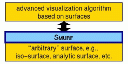Figure 1: SMURF is a C++ interface between visualization algorithms and surface implementation.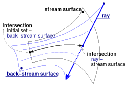Figure 2: Intersecting ray with implicitly specified stream surface.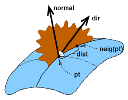Figure 3: Surface-curve traversal.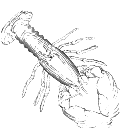Figure 4: Contour display of lobster CT scan -- see also Fig. 5.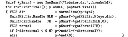Figure 5: Code used for drawing lobster contours depicted in Fig. 4.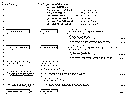Figure 6: SMURF class hierarchy and interface.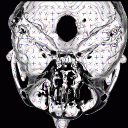Figure 7: Iso-surface computed for ten slices scanned from a human head with curvature crosses.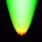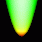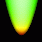Figure 8a, Figure 8b, Figure 8c: Gauss curvature plot using linear (a) and cubic (b) function reconstruction vs. analytic computation (c).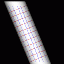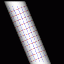Figure 9a, Figure 9b: Quality of curvature calculations depending on the function reconstruction scheme - linear (a) vs. cubic (b) reconstruction.header < abstract < paper < figures < footer

Helwig Löffelmann, last update on October 29, 1998.